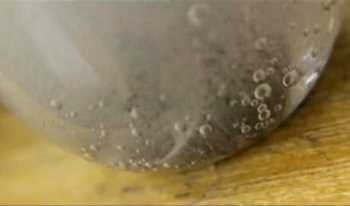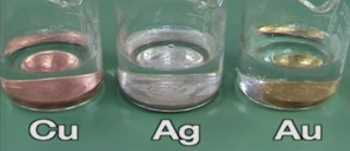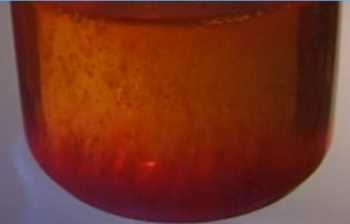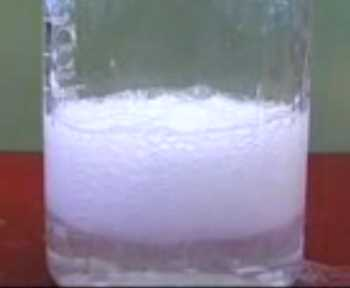Search:

# Reactions of acids

## Neutralisation of bases by $HCl$

### The reaction of chlorhydric acid with sodium hydroxide:Chlorhydric acid (colourless solution) neutralises sodium hydroxide (colourless solution) producing sodium chloride (colourless in solution) and water: $NaOH$ $+$ $HCl$ $\longrightarrow$ $NaCl$ $+$ $H_2O$

### The reaction of chlorhydric acid with magnesium hydroxide:Chlorhydric acid (colourless solution) neutralises magnesium hydroxide (white solid) producing magnesium chloride (colourless in solution) and water: $Mg(OH)_2$ $+$ $2HCl$ $\longrightarrow$ $MgCl_2$ $+$ $2H_2O$

## Reaction of $HCl$ with metals

### The reaction of chlorhydric acid with magnesium:Chlorhydric acid (colourless solution) attacks magnesium producing very quickly magnesium chloride (colourless in solution) and hydrogen (light and colourless gas). $Mg$ $+$ $2HCl$ $\longrightarrow$ $MgCl_2$ $+$ $H_2$

### The reaction of chlorhydric acid with zinc:Chlorhydric acid (colourless solution) attacks zinc producing zinc chloride (colourless in solution) and hydrogen (light and colourless gas). $Zn$ $+$ $2HCl$ $\longrightarrow$ $ZnCl_2$ $+$ $H_2$

### The reaction of chlorhydric acid with iron:Chlorhydric acid (colourless solution) attacks iron producing slowly iron(II) chloride (greenish in solution) and hydrogen (light and colourless gas). $Fe$ $+$ $2HCl$ $\longrightarrow$ $FeCl_2$ $+$ $H_2$

### Action of chlorhydric acid on copper, silver and gold:Chlorhydric acid (colourless solution) doesn't react with copper, silver or gold.

## The reaction of chlorhydric acid with metal oxides

### The reaction of chlorhydric acid with copper(II) oxide:Chlorhydric acid (colourless solution) attacks copper(II) oxide (a black solid) producing copper(II) chloride (greenish-blue in solution) and water: $CuO$ $+$ $2HCl$ $\longrightarrow$ $CuCl_2$ $+$ $H_2O$

### The reaction of chlorhydric acid with iron(III) oxide:Chlorhydric acid (colourless solution) attacks iron(III) oxide (ochred solid) producing iron(III)chloride (ochred in solution) and water: $Fe_2O_3$ $+$ $6HCl$ $\longrightarrow$ $2FeCl_3$ $+$ $3H_2O$

## The reaction of chlorhydric acid with carbonates

### The reaction of chlorhydric acid with sodium carbonate:Chlorhydric acid (colourless solution) attacks sodium carbonate (colourless solution) producing sodium chloride (colourless in solution), carbon dioxide (a dense and colourless gas) and water: $Na_2CO_3$ $+$ $2HCl$ $\longrightarrow$ $2NaCl$ $+$ $CO_2$ $+$ $H_2O$

### The reaction of chlorhydric acid with calcium carbonate:Chlorhydric acid (colourless solution) attacks calcium carbonate (a white solid, in shells or in the limestone of rocks) producing calcium chloride (colourless in solution), carbon dioxide (a dense and colourless gas) and water: $CaCO_3$ $+$ $2HCl$ $\longrightarrow$ $CaCl_2$ $+$ $CO_2$ $+$ $H_2O$

## Reactions of acids

All acids react like chlorhydric acid in dilute solutions:

Acid and base produce salt and water.

Acid and non-noble metal (magnesium, zinc, iron) produce salt and hydrogen.

Acid and metal oxide produce salt and water.

Acid and carbonate produce salt and carbon dioxide and water.

We see:

The hydrogen $H$ atoms of the acids are responsible for their caracteristic reactions!